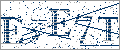>>

Login or Register   Integers - Introduction
 New User RegisterLogin Member: Email: Password:
 Site SearchAdvance Search

Other animationsIntegers - Arithmetic Operations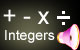Animation product List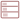Row format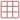Grid formatList formatOverview(For age - group : 11 - 16 )

Fully animated ‘Introduction to integers’ covers the concept using real life examples. This will help you unserstand and apply the concept of positive and negative numbers.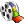Product - Animation
 Size/Duration Subscribe Preview / Trailer Size (KB) 14400 US\$    1.50 Rs.118.50  Check price in your currency Subscription Days = 30 Watch a preview (opens in separate window) Duration (hr:min:sec) 1:13:0 Add To Cart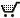This animation has audio.Category : Math Integer Type : Animation with sound Animation Type : Regular Total animation length: 73 minutes The animation covers: What are natural numbers? What are whole numbers? What is a set? What is union of sets? Introduction to integers What are these new negative numbers? What are positive numbers? What are the uses of negative numbers? Representing integers on a number line What do we get from the number line? Why do bigger negative numbers are said to be smaller? History on number line Opposite integer or additive inverse Comparing integers How to compare numbers on a number line? What is an absolute value? Quiz section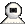Snapshots
 Watch combined previewWatch various movie clips related to integers. These are 1) Absolute value of an integer 2) Arithmetic operations of Integers 3) BODMAS Rule 4) Introduction to Integers 5) Number line - Representing History 6) Introduction to Natural and Whole Numbers 7) Representing Integers on Number line 8) Quiz on Integers. Click on the above thumbnails to go to the movie.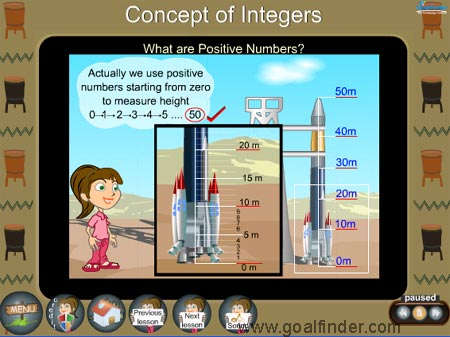Introduction to integers is explained through real life examples and situations, the concept of positive and negative numbers is brought out clearly through these examples.This is like an animated math tutorial but with entertainment and stories to make learning integers a fun. We have provided many examples to clarify doubts in integers.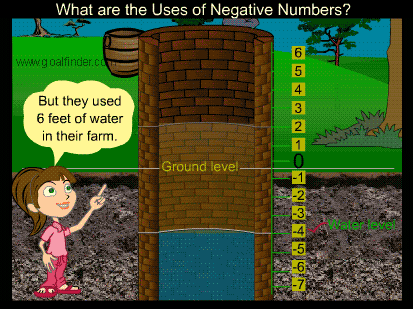Janet explains the concept of negative numbers through the example of a well.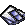Details of the animation/ movie /software

Introduction to Integers with real life examples is the basis of the animation.

The animation explains the concept of natural numbers and whole numbers using real life examples. What are the natural numbers or positive integers? All numbers that are greater than zero are called as natural numbers. Example: 1, 2, 3, 4, 5... and whole numbers by addition of zero to the natural numbers. Example 0, 1, 2...

The concept of set and union of set is explained using examples.

Introduction to integers and positive and negative numbers are supported with more examples. Positive integers means things associated with increasing, ascending, gain etc while decreasing, descending, loss etc denote negative integers.

Another example introduces representing integers on a number line, rules are needed for understanding how to write integers on a number line, for example : An integer is always larger than one on its left.

Then the concept of additive inverse is brought out through number line and real life examples. Example +31 and -31.

Comparing integers through number line and absolute value are also covered.

The animation covers the introduction to integers using real life examples; a quiz section with detailed solution also checks your understanding.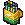Related Products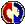Buyers Feedback:Give your comments